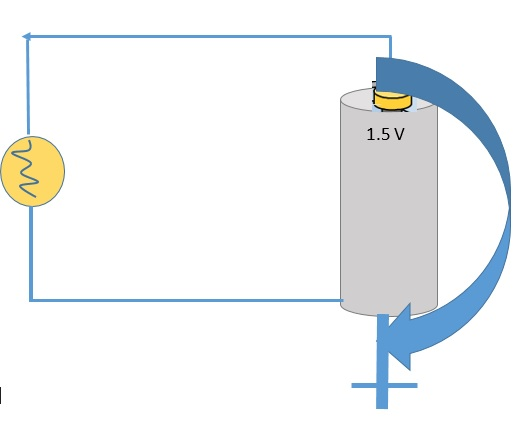# Introduction to Ohm's Law

A battery is the prime mover of charges and source of voltage in an electric circuit.
How much current exists depends not only on the voltage but also on the electrical resistance (R) and the conductor (such as metal wire) which facilitates the flow of charge.

Important characteristics of the resistance:
• The resistance of a wire depends both on the thickness and length of the wire
• (and on other factors like conductivity).
• Thick wires have less resistance than thin wires; long wires have more resistance than short wires.
• Electrical resistance (R) is measured in units called ohms.
• The Greek letter omega, Ω, is used as the symbol for the ohm.
Relationship between the current (I), the voltage (V or ε), and the resistance (R) was summarized by George Simon Ohm in his Ohm’s Law:
Current = Voltage / Resistance or I = ε / R

Example:
Assume that we know the resistance of a given part of a circuit and the applied voltage,
and we want to calculate the current drawn in the circuit.

See the figure below and let's assume a 1.5-V battery connected to a light bulb with a resistance of 30 ohms.Assume also that the resistance of the battery is zero.
Question-1: Find the current using Ohm’s Law:

Solution:
I = 1.5 V / 30 Ω = 0.05 A = 50 mA

Now, let's consider that the battery has an internal resistance equal to 3 Ω.
Question-2: What is the total resistance of the circuit?
Solution:
Total resistance = 30 Ω + 3 Ω = 33 Ω

Question-3: What is the total current in the circuit?
Solution:
Total current I = ε / R = 1.5 V / 33 Ω = 0.045 A = 45 mA.

Question-4: Calculate the voltage difference across the light bulb:
Solution:
Δε = IR = 0.045 A × 30 Ω = 1.36 A

Hence, If we measure the voltage difference across the battery or the light bulb, it would be 1.36 V.

### Exercise 1

Exercise-1 on Ohm's Law

Check your answers here: Solution to Exercise-1 on Ohm's Law

### Exercise 2

Exercise-2 on Ohm's Law

Check your answers here: Solution to Exercise-2 on Ohm's Law

### Exercise 3

Exercise-3 on Ohm's Law

Check your answers here: Solution to Exercise-3 on Ohm's Law

For more details, please contact me here.
Date of last modification: Summer , 2019